## Cross Reference Down

#### Janvresse, É (2012). Quelques contributions aux probabilités eta la théorie ergodique. Document de synthèse présenté pour l’Habilitation à Diriger des Recherches, l’université de Rouen. FRE

This work cites the following items of the Benford Online Bibliography:

 Boyle, J (1994). An Application of Fourier Series to the Most Significant Digit Problem. American Mathematical Monthly 101(9), pp. 879-886. ISSN/ISBN:0002-9890. DOI:10.2307/2975136.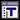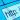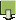Diaconis, P (1977). Examples of the theory of infinite iteration of summability methods. Canadian Journal of Mathematics 29(3), pp. 489-497. DOI:10.4153/CJM-1977-053-1.Duncan, RL (1969). Note on the initial digit problem. Fibonacci Quarterly 7(5), pp. 474-475.Flehinger, BJ (1966). On the Probability that a Random Integer has Initial Digit A. American Mathematical Monthly 73(10), pp. 1056-1061. ISSN/ISBN:0002-9890. DOI:10.2307/2314636.Giuliano, R and Janvresse, E (2010). A unifying probabilistic interpretation of Benford's Law. Uniform Distribution Theory 5(2), pp. 169-182. ISSN/ISBN:1336-913X.Hill, TP (1995). Base-Invariance Implies Benford's Law. Proceedings of the American Mathematical Society 123(3), pp. 887-895. ISSN/ISBN:0002-9939. DOI:10.2307/2160815.Jang, D, Kang, JU, Kruckman, A, Kudo, J and Miller, SJ (2009). Chains of distributions, hierarchical Bayesian models and Benford's Law. Journal of Algebra, Number Theory: Advances and Applications 1(1), pp. 37-60.Janvresse, E and de la Rue, T (2004). From Uniform Distributions to Benford’s Law. Journal of Applied Probability 41(4), pp. 1203-1210. ISSN/ISBN:0021-9002.Janvresse, É and de la Rue, T (2012). Averaging along Uniform Random Integers. Uniform Distribution Theory 7(2), pp. 35–60.Knuth, DE (1997). The Art of Computer Programming. pp. 253-264, vol. 2, 3rd ed, Addison-Wesley, Reading, MA.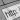Miller, SJ and Nigrini, MJ (2008). The Modulo 1 Central Limit Theorem and Benford's Law for Products. International Journal of Algebra 2(3), pp. 119 - 130.Newcomb, S (1881). Note on the frequency of use of the different digits in natural numbers. American Journal of Mathematics 4(1), pp. 39-40. ISSN/ISBN:0002-9327. DOI:10.2307/2369148.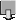Pinkham, RS (1961). On the Distribution of First Significant Digits. Annals of Mathematical Statistics 32(4), pp. 1223-1230. ISSN/ISBN:0003-4851.Raimi, RA (1976). The First Digit Problem. American Mathematical Monthly 83(7), pp. 521-538. ISSN/ISBN:0002-9890. DOI:10.2307/2319349.